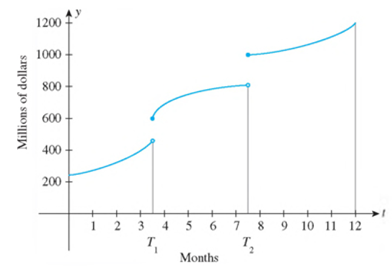Chapter 2.5, Problem 64E

Chapter
Section
Textbook Problem

AILING FINANCIAL INSTITUTIONS Franklin Savings and Loan acquired two ailing financial institutions in 2010. One of them was acquired at time t = T1, and the other was acquired at time t = T2 (t = 0 corresponds to the beginning of 2010). The following graph shows the total amount of money on deposit with Franklin. Explain the significance of the discontinuities of the function at T1 and T2.To determine

To explain: The significance of discontinuities of the function at T1 and T2 .

Explanation

The graph in Figure (1) shows the total amount of money on deposit in Millions of dollars for different months, and the interval of 12 months has been divided into three parts.

Figure (1)

In first part, from time t=0 to t=T1 which is a period of three and half months, the company worked as a single company and raised its assets from 250milliondollars to 450milliondollars .

Between the month of March and April 2010 , one company was acquired and the assets of the company got enhanced from 450milliondollars to 600milliondollars

Still sussing out bartleby?

Check out a sample textbook solution.

See a sample solution

The Solution to Your Study Problems

Bartleby provides explanations to thousands of textbook problems written by our experts, many with advanced degrees!

Get Started

Find f g h. 40. f(x) = |x 4|, g(x) = 2x, h(x)=x

Single Variable Calculus: Early Transcendentals, Volume I

Convert the expressions in Exercises 6584 to power form. 35x2

Finite Mathematics and Applied Calculus (MindTap Course List)

A population of N = 15 scores has SX = 120. What is the population mean?

Statistics for The Behavioral Sciences (MindTap Course List)

74. Factor as indicated:

Mathematical Applications for the Management, Life, and Social Sciences

Find each product: (r12)2

Elementary Technical Mathematics

Proof Use the definition of infinite limits at infinity to prove that limxx3=

Calculus: Early Transcendental Functions (MindTap Course List)